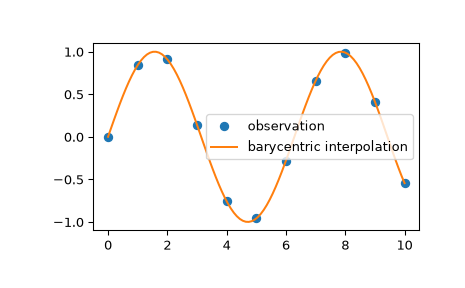# scipy.interpolate.barycentric_interpolate¶

scipy.interpolate.barycentric_interpolate(xi, yi, x, axis=0)[source]

Convenience function for polynomial interpolation.

Constructs a polynomial that passes through a given set of points, then evaluates the polynomial. For reasons of numerical stability, this function does not compute the coefficients of the polynomial.

This function uses a “barycentric interpolation” method that treats the problem as a special case of rational function interpolation. This algorithm is quite stable, numerically, but even in a world of exact computation, unless the x coordinates are chosen very carefully - Chebyshev zeros (e.g., cos(i*pi/n)) are a good choice - polynomial interpolation itself is a very ill-conditioned process due to the Runge phenomenon.

Parameters
xiarray_like

1-D array of x coordinates of the points the polynomial should pass through

yiarray_like

The y coordinates of the points the polynomial should pass through.

xscalar or array_like

Points to evaluate the interpolator at.

axisint, optional

Axis in the yi array corresponding to the x-coordinate values.

Returns
yscalar or array_like

Interpolated values. Shape is determined by replacing the interpolation axis in the original array with the shape of x.

BarycentricInterpolator

Bary centric interpolator

Notes

Construction of the interpolation weights is a relatively slow process. If you want to call this many times with the same xi (but possibly varying yi or x) you should use the class BarycentricInterpolator. This is what this function uses internally.

Examples

We can interpolate 2D observed data using barycentric interpolation:

>>> import matplotlib.pyplot as plt
>>> from scipy.interpolate import barycentric_interpolate
>>> x_observed = np.linspace(0.0, 10.0, 11)
>>> y_observed = np.sin(x_observed)
>>> x = np.linspace(min(x_observed), max(x_observed), num=100)
>>> y = barycentric_interpolate(x_observed, y_observed, x)
>>> plt.plot(x_observed, y_observed, "o", label="observation")
>>> plt.plot(x, y, label="barycentric interpolation")
>>> plt.legend()
>>> plt.show()#### Previous topic

scipy.interpolate.KroghInterpolator.derivatives

#### Next topic

scipy.interpolate.krogh_interpolate